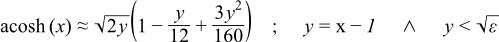#Boost C++ Libraries

...one of the most highly regarded and expertly designed C++ library projects in the world.

This is the documentation for an old version of boost. Click here for the latest Boost documentation.

#### acosh

```#include <boost/math/special_functions/acosh.hpp>
```

```template<class T>
calculated-result-type acosh(const T x);

template<class T, class Policy>
calculated-result-type acosh(const T x, const Policy&);
```

Computes the reciprocal of (the restriction to the range of `[0;+∞[`) the hyperbolic cosine function, at x. Values returned are positive.

If x is in the range `]-∞;+1[` then returns the result of domain_error.

The return type of this function is computed using the result type calculation rules: the return type is `double` when T is an integer type, and T otherwise.

The final Policy argument is optional and can be used to control the behaviour of the function: how it handles errors, what level of precision to use etc. Refer to the policy documentation for more details.##### Accuracy

Generally accuracy is to within 1 or 2 epsilon across all supported platforms.

##### Testing

This function is tested using a combination of random test values designed to give full function coverage computed at high precision using the "naive" formula:along with a selection of sanity check values computed using functions.wolfram.com to at least 50 decimal digits.

##### Implementation

For sufficiently large x, we can use the approximation:For x sufficiently close to 1 we can use the approximation:Otherwise for x close to 1 we can use the following rearrangement of the primary definition to preserve accuracy:Otherwise the primary definition is used: